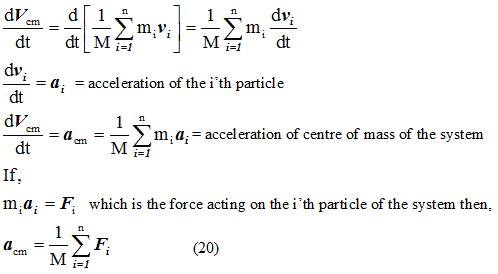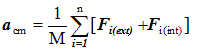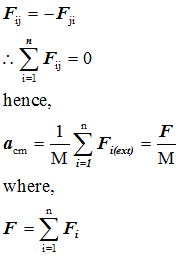# Acceleration of center of mass

## (6) Acceleration of center of mass

• Differentiating equation 7 we get• If miai = Fi which is the force acting on the i'th particle of the system then• Net force acting on the i'th is
Fi=Fi(ext)+Fi(int)
Here internal force is produced due to the mutual interaction between the particles of the system. Therefore, from newton's third law of motionis the total external force acting on the system since internal force on the system because of mutual interaction between the particles of the system become equal to zero because of the action reaction law.
• Hence from equation 23 it is clear that the centre of mass of the system of particles moves a if the whole mass of the system were concentrated at it. This result holds whether the system is a rigid body with particles in fixed position or system of particles with internal motions.

Watch this tutorial for information on How to solve Center of Mass problems